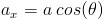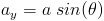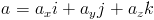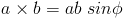# Summary of Chapter 3: Vectors

Scalars and Vectors Scalars are measurements that do not have direction, like temperature. Scalars follow the rules of Regular Algebra. Vectors contain both magnetude and direciton and follow the rules of vector algebra.

Adding Vectors Geometrically Two vectors may be added by drawing them at a common scale head to tail. the vector connecting the final head and tail is the sum of the two vectors. To subtract one vector from another, reverse the direction of the vector you wish to subtract.

components of a Vector To find the x component of a vector, place the tail of the vector at the oragin, and measure the angle (θ) from the x axis. if the magnetude of the vector is given by a then the x component can by found by:The same true of the component along the y axis (use the same angle):Unit Vector Notation Vectors can be written in unit vector notation. Unit vectors are "i" "j" and "k". each is a vector with a magnetude of 1 along the x, y and z axis respectively. By multiplying these vectors by the vector magnetude along the corresponding axis the vector can be written in unit vector notation.unit vectors along the same axis can be added

Product of a Scalar and Vector The product of a scalar and a vector is a new vector with identical direciton to the first, and magnetude equal to the product of the magnetude of the vector and the magnetude of the scalar. To divide, multiply the vector by 1/s where s is the magnetude of the scalar.

Vector Product The product of two vectors is the cross product of the two. The magnetude of the resulting vector is given by:Where the sin of the angle between the two vectors is taken. The resulting vector of this operation is perpendicular to the plane according to the right hand rule.